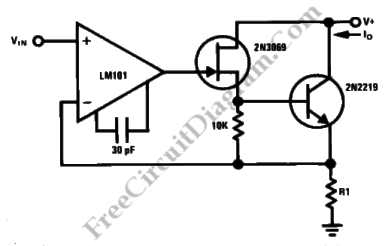# Precision Current SinkThis a a simple circuit for precision current sink. This circuit uses two kinds of transistor, 2N2219 bipolar transistor and 2N3069 JFET because both of them have inherently high output impedance. Here is the circuit :To provide feedback to the LM101 op amp, this circuit uses R1 as a current sensing resistor. The true current sink nature of this circuit is enhanced because the op amp provides a large amount of loop gain for negative feedback.
The output current is given by :
Io = Vin/R1
with Vin > 0 V
The 2N2219 and the 10K resistor may be eliminated, for small current values, if the JFET’s source is connected to R1. [Source: National Semiconductor Application Note]